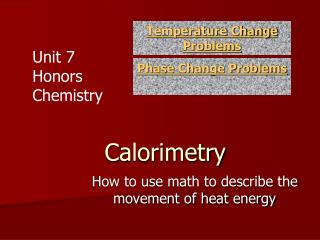DownloadDownload PresentationCalorimetry

# Calorimetry

Download Presentation## Calorimetry

- - - - - - - - - - - - - - - - - - - - - - - - - - - E N D - - - - - - - - - - - - - - - - - - - - - - - - - - -
##### Presentation Transcript

1. Temperature Change Problems Unit 7 Honors Chemistry Phase Change Problems Calorimetry How to use math to describe the movement of heat energy

2. Energy Conversions • Heat is a specific type of energy that can be measured in different ways. • The SI unit for heat is Joules • 4.184 Joules = 1 calorie (this will be given) • 1000 calories = 1 kilocalorie • 1000 Joules = 1 kiloJoule

3. Heat Conversions • How many joules are in 130 calories? • How many calories are in 50 Joules? 4.184 Joules 130 calories = 543.92 Joules (= 540 J (Sig figs!) 1 calorie 50 Joules 1 calorie = 11.95 calories 4.184 Joules

4. Heat Conversions • How many kilojoules are in 130 Calories? 1 kJ 4.184 Joules 130 Calories 1000J 1 Calorie = 0.54 KiloJoules

5. Calorimetry • Allows us to calculate the amount of energy required to heat up a substance or to make a substance change states. • Molar Heat of Fusion (Hf)— The heat absorbed by one mole of a substance when changing from a solid to a liquid. • For water, it = 6.0 kiloJoules/mole • or 334 Joules/gram (specific heat of fusion) • Heat of solidification is opposite of heat of fusion (heat is released).

6. Molar Heat of Vaporization (Hv)— The heat absorbed by one mole of a substance when changing from a liquid to a gas. • For water, it = 40.7 kiloJoules/mole. or 2260 Joules/gram (specific heat of vaporization • Heat of condensation is the opposite of heat of vaporization (heat is released) • Every pure substance will have a unique Molar heat of fusion (Hf) or vaporization (Hv)

7. Heat Required For a Phase Change • Heat Absorbed or Released = q • For Melting or Freezing use the following: • For Vaporization or Condensation use the following: q = (moles) x Molar Heat Fusion q = (moles) x Molar Heat vaporization

8. Calculating Heat Required To Change State • Example #1: How much heat is needed to melt 56.0 grams of ice into liquid (the molar heat of fusion for ice is 6.0 kJ/mol)? • 56.0 g 1 mole H2O 6.0 kJ = 18.0 g 1 mole • = 18.7 kJ will be absorbed q = (moles) x (Hf)

9. Example #2 • How much heat energy in kJ will be released when 200grams steam condenses back to a liquid water? • Hv = 40.7kJ/mol q = (moles) x (Hv) 200gram 1 mole 40.7 kJ 18gram 1 mole = 452 kJ released Or -452kJ

10. Heating a Substance with No Phase Change • Specific Heat Capacity--The amount of energy required to raise one gram of a substance one degree Celcius. Water’s Specific Heat (as a liquid) Cp= 4.184 Joules/gram oC *Every pure substance will have its own unique specific heat for every phase!

11. Heating a Substance with No Phase Change • When you see an increase in the temperature of a sample, the heat is being added to raise the temperature • How much the temperature increases is based upon the heat capacity (Cp) and the massof your sample • The higher the heat capacity number, the longer it takes to heat a substance up and the longer the substance holds on to the heat.

12. Energy to Change Temperature q = (mass) ( Cp) ( T ) Change in Temperature Tfinal – Tinitial In OCelcius Heat Measured in Joules Specific Heat Capacity Mass In grams

13. Example #3 m How much energy is needed to heat 80 g of water from 10 oC to 55 oC? Tfinal Tinitial q = mCpΔT = m Cp (Tfinal – Tinitial ) = (80g) ( 4.184 J/g C) (55oC – 10oC) q = 15062 joules Is the energy absorbed or released? Absorbed, because temperature inincreasing Final Answer: 15,062 J = 15.06 kJ absorbed/ endothermic

14. Example #4 m How much energy is needed to cool 150 g of ice from -2 oC to -55 oC? Tfinal Tinitial q = mCpΔT = m Cp (Tfinal – Tinitial ) = (150g) ( 2.06 J/g C) (-55oC – -2oC) q = - 16377 joules Is the energy absorbed or released? Released, because temperature indecreasing Final Answer: - 16377 J = -16.3 kJ released/exothermic

15. Heat Problem Road Map q = (moles)Hv q = (moles)Hf Gas Heats Vaporization or Condensation Melting or Freezing Liquid Heats q = mCpΔT Solid Heats * Add each individual energies (in kJ) together for total heat energy required for multistep problems (up to 5 steps max!)

16. This problem requires two steps. Since water is solid ice at 0oC, we need to melt the ice and then heat it up to 50oC. • Example #5 -How much energy in kJ is needed to change 150grams of ice from 0oC to 50oC? Step 1 – Calculate heat required to melt 150grams ice 150g 1 mole 6.0 kJ = 50 kJ 18grams 1 mole Step 2 - Calculate heat required to heat liquid water from 0oC to 50oC q = mC T = (150g)(4.184 J/goC)(50oC) = 31380 J  convert to kJ = 31.38kJ *Add both heat values together for your final answer 50 kJ + 31.38kJ = 81.38 kJ heat absorbed.

17. Calorimetry Formula Summary Phase Change • Use Molar Heat constants Melting use q = (moles) x (Hfusion) Vaporize use q = (moles) x (HVaporization) No Phase Change • Use specific heat capacity q = (mass) ( Cp ) ( ΔT )